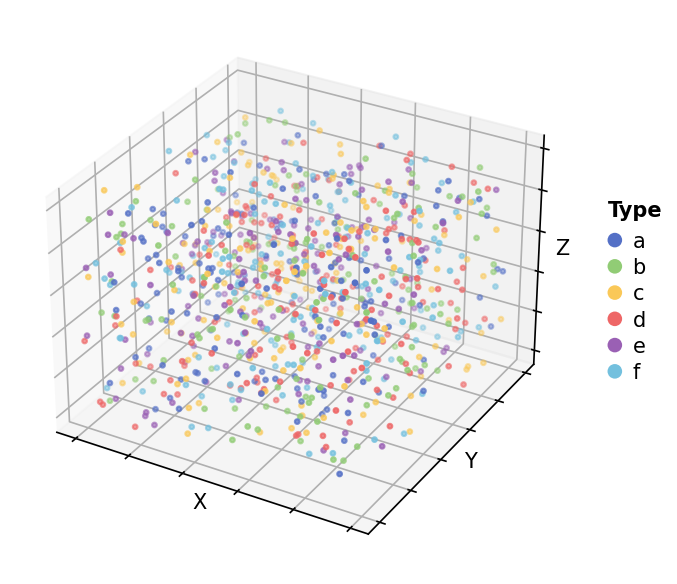# Point map 3D Example (Categorical data)#

Here shows how to draw the point map in 3D

```import numpy as np
import milkviz as mv
```

## First let’s create some random data#

```np.random.seed(0)
xyz = np.random.randint(0, 100, (1000, 3))
types = np.random.choice(list("abcdef"), 1000)
```

## Create the point map#

```mv.point_map(xyz, types=types, legend_kw={"title": "Type"})
``````<Axes3DSubplot: xlabel='X', ylabel='Y', zlabel='Z'>
```

Total running time of the script: ( 0 minutes 0.592 seconds)

Gallery generated by Sphinx-Gallery